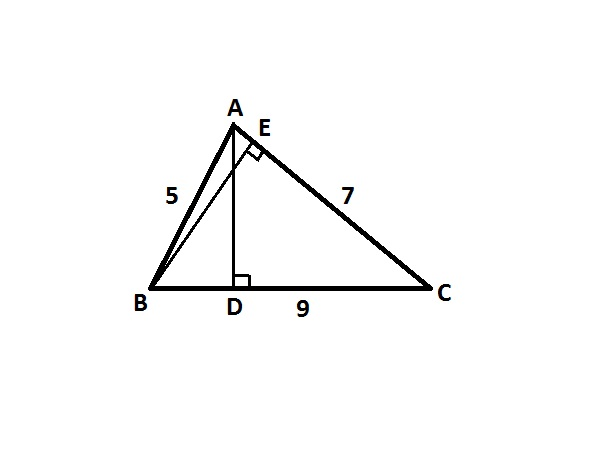# Everyday Triangle ProblemA triangle $\Delta ABC$ has side lengths of $5$, $7$ and $9$ units respectively.

$AD \perp BC$ is drawn. Similarly, $BE \perp AC$ is drawn.

Find the length of $DE$ to 2 decimal places.

Note: The image given is just for reference and not to be scaled. The actual figure may vary.

×